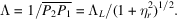International
Tables for
Crystallography
Volume B
Reciprocal space
Edited by U. Shmueli

International Tables for Crystallography (2006). Vol. B, ch. 5.1, p. 543   | 1 | 2 |

## Section 5.1.6.3.2. Amplitudes – Pendellösung

A. Authiera*

aLaboratoire de Minéralogie-Cristallographie, Université P. et M. Curie, 4 Place Jussieu, F-75252 Paris CEDEX 05, France
Correspondence e-mail: authier@lmcp.jussieu.fr

#### 5.1.6.3.2. Amplitudes – Pendellösung

| top | pdf |

We shall assume from now on that the crystal is plane parallel. Two wavefields arrive at any point of the exit surface. Their constituent waves interfere and generate emerging waves in the refracted and reflected directions (Fig. 5.1.6.4). Their respective amplitudes are given by the boundary conditions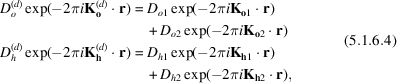where r is the position vector of a point on the exit surface, the origin of phases being taken at the entrance surface.Figure 5.1.6.4 | top | pdf |Decomposition of a wavefield into its two components when it reaches the exit surface.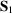and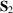are the Poynting vectors of the two wavefields propagating in the crystal belonging to branches 1 and 2 of the dispersion surface, respectively, and interfering at the exit surface.

In a plane-parallel crystal, (5.1.6.4)reduces to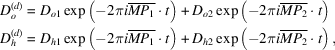where t is the crystal thickness.

In a non-absorbing crystal, the amplitudes squared are of the form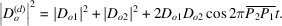This expression shows that the intensities of the refracted and reflected beams are oscillating functions of crystal thickness. The period of the oscillations is called the Pendellösung distance and is